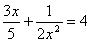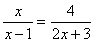Introduction

A rational equation is an equation containing rational expressions.

Examples of rational equations: